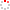NSSC OpenIR  > 空间科学部
 Monte Carlo simulations of a diffusive shock with multiple scattering angular distributions Wang, X.; Yan, Y. H.; Wang, X (reprint author), Chinese Acad Sci, Natl Astron Observ, Key Lab Solar Activ, Beijing 100012, Peoples R China. Department 空间科学部 Source Publication ASTRONOMY & ASTROPHYSICS2011 Volume 530Pages:A92 ISSN 0004-6361 Language 英语 Keyword Acceleration Of Particles Methods: Numerical Shock Waves Abstract Aims. We independently develop a simulation code following the previous dynamical Monte Carlo simulation of the diffusive shock acceleration under the isotropic scattering law during the scattering process, and the same results are obtained. Methods. Since the same results test the validity of the dynamical Monte Carlo method for simulating a collisionless shock, we extend the simulation toward including an anisotropic scattering law for further developing this dynamical Monte Carlo simulation. Under this extended anisotropic scattering law, a Gaussian distribution function is used to describe the variation of scattering angles in the particle's local frame. Results. As a result, we obtain a series of different shock structures and evolutions in terms of the standard deviation values of the given Gaussian scattering angular distributions. Conclusions. We find that the total energy spectral index increases as the standard deviation value of the scattering angular distribution increases, but the subshock's energy spectral index decreases as the standard deviation value of the scattering angular distribution increases.; Aims. We independently develop a simulation code following the previous dynamical Monte Carlo simulation of the diffusive shock acceleration under the isotropic scattering law during the scattering process, and the same results are obtained. Methods. Since the same results test the validity of the dynamical Monte Carlo method for simulating a collisionless shock, we extend the simulation toward including an anisotropic scattering law for further developing this dynamical Monte Carlo simulation. Under this extended anisotropic scattering law, a Gaussian distribution function is used to describe the variation of scattering angles in the particle's local frame. Results. As a result, we obtain a series of different shock structures and evolutions in terms of the standard deviation values of the given Gaussian scattering angular distributions. Conclusions. We find that the total energy spectral index increases as the standard deviation value of the scattering angular distribution increases, but the subshock's energy spectral index decreases as the standard deviation value of the scattering angular distribution increases. Indexed By SCI ; EI Funding Project 中国科学院空间科学与应用研究中心 Document Type 期刊论文 Identifier http://ir.nssc.ac.cn/handle/122/3132 Collection 空间科学部 Corresponding Author Wang, X (reprint author), Chinese Acad Sci, Natl Astron Observ, Key Lab Solar Activ, Beijing 100012, Peoples R China. Recommended CitationGB/T 7714 Wang, X.,Yan, Y. H.,Wang, X . Monte Carlo simulations of a diffusive shock with multiple scattering angular distributions[J]. ASTRONOMY & ASTROPHYSICS,2011,530:A92. APA Wang, X.,Yan, Y. H.,&Wang, X .(2011).Monte Carlo simulations of a diffusive shock with multiple scattering angular distributions.ASTRONOMY & ASTROPHYSICS,530,A92. MLA Wang, X.,et al."Monte Carlo simulations of a diffusive shock with multiple scattering angular distributions".ASTRONOMY & ASTROPHYSICS 530(2011):A92.